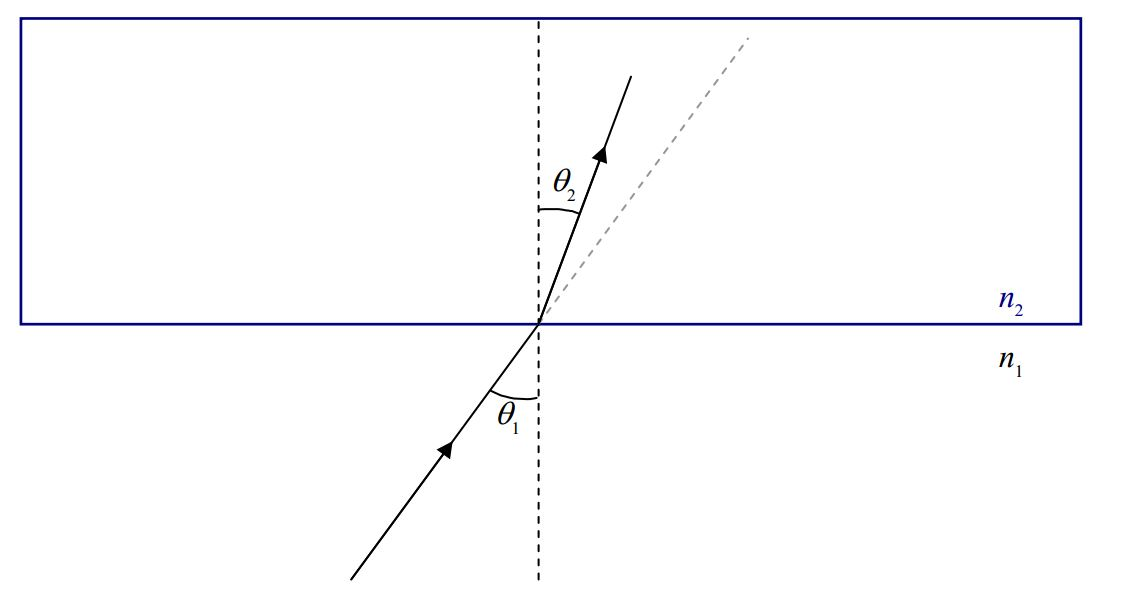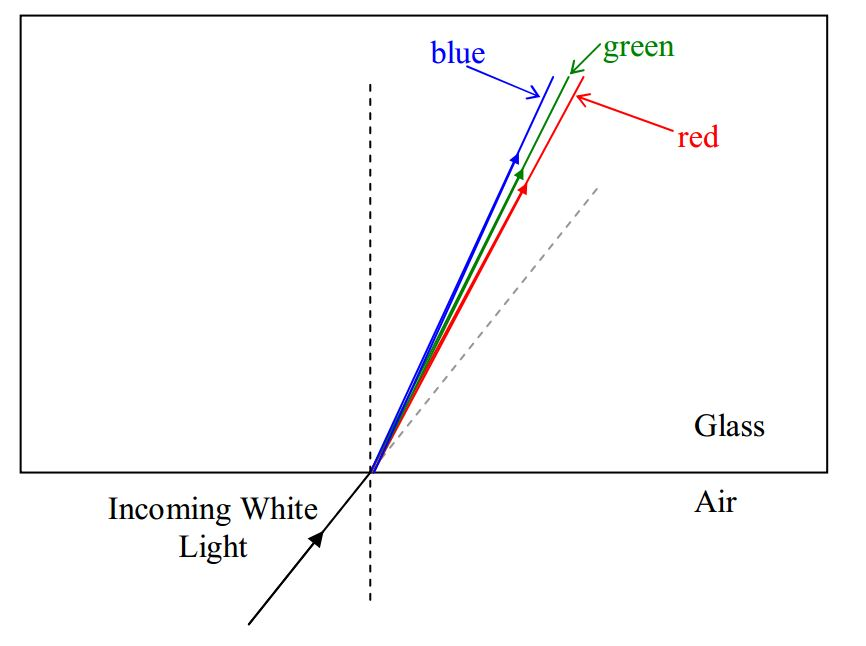# B27: Refraction, Dispersion, Internal Reflection

$$\newcommand{\vecs}{\overset { \rightharpoonup} {\mathbf{#1}} }$$ $$\newcommand{\vecd}{\overset{-\!-\!\rightharpoonup}{\vphantom{a}\smash {#1}}}$$$$\newcommand{\id}{\mathrm{id}}$$ $$\newcommand{\Span}{\mathrm{span}}$$ $$\newcommand{\kernel}{\mathrm{null}\,}$$ $$\newcommand{\range}{\mathrm{range}\,}$$ $$\newcommand{\RealPart}{\mathrm{Re}}$$ $$\newcommand{\ImaginaryPart}{\mathrm{Im}}$$ $$\newcommand{\Argument}{\mathrm{Arg}}$$ $$\newcommand{\norm}{\| #1 \|}$$ $$\newcommand{\inner}{\langle #1, #2 \rangle}$$ $$\newcommand{\Span}{\mathrm{span}}$$ $$\newcommand{\id}{\mathrm{id}}$$ $$\newcommand{\Span}{\mathrm{span}}$$ $$\newcommand{\kernel}{\mathrm{null}\,}$$ $$\newcommand{\range}{\mathrm{range}\,}$$ $$\newcommand{\RealPart}{\mathrm{Re}}$$ $$\newcommand{\ImaginaryPart}{\mathrm{Im}}$$ $$\newcommand{\Argument}{\mathrm{Arg}}$$ $$\newcommand{\norm}{\| #1 \|}$$ $$\newcommand{\inner}{\langle #1, #2 \rangle}$$ $$\newcommand{\Span}{\mathrm{span}}$$$$\newcommand{\AA}{\unicode[.8,0]{x212B}}$$

When we talked about thin film interference, we said that when light encounters a smooth interface between two transparent media, some of the light gets through, and some bounces off. There we limited the discussion to the case of normal incidence. (Recall that normal means perpendicular to and normal incidence is the case where the direction in which the light is traveling is perpendicular to the interface.) Now we consider the case in which light shining on the smooth interface between two transparent media, is not normally incident upon the interface. Here’s a “clean” depiction of what I’m talking about:and here’s one that’s all cluttered up with labels providing terminology that you need to know:As in the case of normal incidence, some of the light is reflected and some of it is transmitted through the interface. Here we focus our attention on the light that gets through.Experimentally we find that the light that gets through travels along a different straight line path than the one along which the incoming ray travels. As such, the transmitted ray makes an angle $$\theta_2$$ with the normal that is different from the angle $$\theta_1$$ that the incident ray makes with the normal.

The adoption of a new path by the transmitted ray, at the interface between two transparent media is referred to as refraction. The transmitted ray is typically referred to as the refracted ray, and the angle $$\theta_2$$ that the refracted ray makes with the normal is called the angle of refraction. Experimentally, we find that the angle of refraction $$\theta_2$$ is related to the angle of incidence $$\theta_1$$ by Snell’s Law:

$n_1 \sin\theta_1=n_2 \sin\theta_2 \label{27-1}$

where:

• $$n_1$$ is the index of refraction of the first medium, the medium in which the light is traveling before it gets to the interface,
• $$\theta_1$$ is the angle that the incident ray (the ray in the first medium) makes with the normal,
• $$n_2$$ is the index of refraction of the second medium, the medium in which the light is traveling after it goes through the interface, and,
• $$\theta_2$$ is the angle that the refracted ray (the ray in the second medium) makes with the normal.

## Dispersion

On each side of the equation form of Snell’s law we have an index of refraction. The index of refraction has the same meaning as it did when we talked about it in the context of thin film interference. It applies to a given medium. It is the ratio of the speed of light in that medium to the speed of light in vacuum. At that time, I mentioned that different materials have different indices of refraction, and in fact, provided you with the following table:

Medium Index of Refraction
Vacuum 1
Air 1.00
Water 1.33
Glass(Depends on the kind of glass. Here is one typical value.) 1.5

What I didn’t mention back then is that there is a slight dependence of the index of refraction on the wavelength of the visible light, such that, the shorter the wavelength of the light, the greater the index of refraction. For instance, a particular kind of glass might have an index of refraction of 1.49 for light of wavelength 695 nm (red light), but an index of refraction that is greater than that for shorter wavelengths, including an index of refraction of 1.51 for light of wavelength 405 nm (blue light). The effect in the case of a ray of white light traveling in air and encountering an interface between air and glass is to cause the different wavelengths of the light making up the white light to refract at different angles.This phenomena of white light being separated into its constituent wavelengths because of the dependence of the index of refraction on wavelength, is called dispersion.

This page titled B27: Refraction, Dispersion, Internal Reflection is shared under a CC BY-SA 2.5 license and was authored, remixed, and/or curated by Jeffrey W. Schnick via source content that was edited to the style and standards of the LibreTexts platform; a detailed edit history is available upon request.